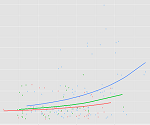### Segmented Regression for Non-Constant Relationships

January 8th, 2018 byWhen you put a continuous predictor into a linear regression model, you assume it has a constant relationship with the dependent variable along the predictor’s range. But how can you be certain? What is the best way to measure this?

And most important, what should you do if it clearly isn’t the case?

Let’s explore a few options for capturing a non-linear relationship between X and Y within a linear regression (yes, really). (more…)

### How to Combine Complicated Models with Tricky Effects

July 22nd, 2011 by

Need to dummy code in a Cox regression model?Interpret interactions in a logistic regression?This is where statistical analysis starts to feel really hard. You’re combining two difficult issues into one.

You’re dealing with both a complicated modeling technique at Stage 3 (survival analysis, logistic regression, multilevel modeling) and tricky effects in the model (dummy coding, interactions, and quadratic terms).

The only way to figure it all out in a situation like that is to break it down into parts.  (more…)

### Regression Models:How do you know you need a polynomial?

April 3rd, 2009 by

A polynomial term–a quadratic (squared) or cubic (cubed) term turns a linear regression model into a curve.  But because it is X that is squared or cubed, not the Beta coefficient, it still qualifies as a linear model.  This makes it a nice, straightforward way to model curves without having to model complicated non-linear models.

But how do you know if you need one–when a linear model isn’t the best model? (more…)